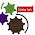## Saturday, 9 January 2016

### Harmonic Progression-Write A Program To Find SUM of Infinite HP.- C Programming Examples

As we have started C Programming coding with Example Series, We have published last posts "Write A Program To Find SUM of AP" and "Write A Program To Find SUM of GP Sequence". We have chosen to start with mathematical series and in this post you will find some basic idea and some useful concepts about Harmonic Progression (H.P.).You can download C Codes below.Please let us know your feedback.

Common C Questions :TURBO C/C++
Integrated Environment : TURBO C/C++ - Getting Started
TURBO C/C++: Installer and Installation
C Language Programming With Examples-INDEX

Harmonic Progression/Sequence Step-wise Video Tutorial(See C- Codes Below ):

In mathematics, a Harmonic Progression (or harmonic sequence) is a progression formed by taking the reciprocals of an arithmetic progression. In other words, it is a sequence of the form.
where −a/d is not a natural number and k is a natural number.

Equivalently, a sequence is a harmonic progression when each term is the harmonic mean of the neighboring terms.It is not possible for a harmonic progression (other than the trivial case where a = 1 and k = 0) to sum to an integer. The reason is that, necessarily, at least one denominator of the progression will be divisible by a prime number that does not divide any other denominator.

A general H.P. is 1/a + 1/(a + d) + 1(a + 2d) + ...

nth term of an H.P. = 1/[a +(n -1)d]

Three numbers a, b, c are in H.P. if 1/a, 1/b, 1/c are in A.P.
i.e. if 1/a + 1/c = 2/b
i.e. if b= 2ac/(a + c)
Thus the H.M. between a and b is H = 2ac/(a + c).(Source)

Write A Program In C To Find SUM of Harmonic Progression-Series- Sequence:C Codes are written and tested in TuboC/C++ IDE as you can see in Video:

/*
*Write a Program in C to Find the Sum of H.P Series
*/
#include <stdio.h>

void main()
{
int n;   //Integer Declaration
float i, sum, term;   //Float Declaration

printf("\n\n1 + 1 / 2 + 1 / 3 +......+1 / n \n"); //Show the format of the series to the user
printf("Enter the value of n \n");
scanf("%d", &n);     // %d for accepting integer value
sum = 0;
for (i = 1; i <= n; i++)    //For loop for n Times
{
term = 1 / i;
sum = sum + term;  //Adding each individual term in each looping
}
printf("the Sum of H.P Series is = %f", sum);    //Print result on the screen Float Type
delay(5000);    // To Show the result to the user
}

1.Very interesting blog. A lot of blogs I see these days don't really provide anything that attract others, but I'm most definitely interested in this one. Just thought that I would post and let you know.

1.Thanks for your encouraging Words.We hope you will keep giving us your valuable feedback.

2.You might want to format that code, it's easier to read.

1.@ionut Thanks sir.
We will try our best to keep formatting in mind next time.
May be we will try putting it in iframe. Lets see.Thanks for your valuable inputs.

3.mcafee.com/activate Online Help – Call 1-844-584-0060 Tollfree. Step by Step guide for McAfee Activation, Download & complete installation online. www.mcafee.com/activate or Mcafee activate

4.Very informative, keep posting such smart articles, it extraordinarily helps to understand relating to things.